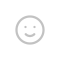## 输出预览

Tell me your X position:
10 # 我输入的值
0 # 我输入的值

Original X position is 10.0
Original Y position is 0.0.
Original position is (10.0,0.0).
New X position is 12.0
New Y position is -1.0
New position is ((12.0, -1.0))

Introduction rules
There are four cases in total
Add 1 X position and reduce 2 Y position
Add 2 X position and reduce 1 Y position
Add 4 X position and add 6 Y position

## 全部代码

############################
### Date 2021 October 1  ###
### Author Magneto       ###
### Name 坐标移动         <——>
### Random True          ###
### Language Python      ###
############################
# 引入 random 模块
import random
spead_0 = ['slow', 'medium', 'fast', 'veryfast']
# 用户输入X坐标
your_x_position = input()
# 用户输入Y坐标
your_y_position = input()
# 转化X坐标
x_position = float(your_x_position)
# 转化Y坐标（+1为初始位置）
y_position = float(your_y_position)
# 输出初始坐标值
print(f"\nOriginal X position is {x_position}"
f"\nOriginal Y position is {y_position}."
f"\nOriginal position is ({x_position},{y_position}).")
# if-elif-else语句
# 定义slow
# 算法
x_increment = x_position + 1
y_increment = y_position - 2
# 定义medium
# 算法
x_increment = x_position + 2
y_increment = y_position - 1
# 定义fast
# 算法
x_increment = x_position + 3
y_increment = y_position + 3
# 定义其它内容，假定其它内容速度为极快，达到仪表显示上限
else:
# 算法
x_increment = x_position + 4
y_increment = y_position + 6
# 输出移动后坐标
print(f"New X position is {x_increment}"
f"\nNew Y position is {y_increment}"
f"\nNew position is ({x_increment,y_increment})")
# 内容介绍定义
alien = {
'slow': 'Add 1 X position and reduce 2 Y position',
'medium': 'Add 2 X position and reduce 1 Y position',
}
print("\nIntroduction rules")
# 字符替换和介绍类型总数
if len(alien) == 4:
# 字符替换
The_number = 'four'
# 介绍类型总数
print(f"There are {The_number} cases in total")
# 其他类型
else:
# 输出空值
print("NULL")
# for语句输出
print(f"{position}")

## 代码分析

#### random模块

# 引入 random 模块
import random
spead_0 = ['slow', 'medium', 'fast', 'veryfast']
# 输出数值
print(f"当前速度是{spead_random}")

eg:

#### 人为输入

# 用户输入X坐标
your_x_position = input()
# 用户输入Y坐标
your_y_position = input()

a = input("Tell me your X positison:")

eg:
Tell me your X position:0    # 0是我输入的值

#### 解决运算问题

a = input("Number is ")
b = a + 3
print(f"Now your number is {b}")

b = a + 3
TypeError: can only concatenate str (not "int") to str

a = input("Number is ")
b= float(a)
c = b + 3
print(f"Now your number is {c}")

eg:
Number is 1
4.0

# 转化X坐标
x_position = float(your_x_position)
# 转化Y坐标（+1为初始位置）
y_position = float(your_y_position)

#### 输出初始坐标

print(f"\nOriginal X position is {x_position}"
f"\nOriginal Y position is {y_position}."
f"\nOriginal position is ({x_position},{y_position}).")

print() 中，我们不必书写 \n 对文字进行换行，可以直接 回车 以换行。当然 \n 的写法也能生效。

print("这是一段文字")

eg:

message = 文字
print(f"这是一段{message}")

eg:

if-elif-else语句

# if-elif-else语句
# 定义slow
# 算法
x_increment = x_position + 1
y_increment = y_position - 2
# 定义medium
# 算法
x_increment = x_position + 2
y_increment = y_position - 1
# 定义fast
# 算法
x_increment = x_position + 3
y_increment = y_position + 3
# 定义其它内容，假定其它内容速度为极快，达到仪表显示上限
else:
# 算法
x_increment = x_position + 4
y_increment = y_position + 6

# if-elif-else语句                    #
# 定义slow                            #
# 算法                            #
x_increment = x_position + 1      # x_increment(新建量)的值是x_position的值+1
y_increment = y_position - 2      # y_increment(新建量)的值是x_position的值-2
# 定义medium                          #
# 算法                            #
x_increment = x_position + 2      # x_increment的值是x_position的值+2
y_increment = y_position - 1      # y_increment的值是x_position的值-1
# 定义fast                            #
# 算法                            #
x_increment = x_position + 3      # x_increment的值是x_position的值+3
y_increment = y_position + 3      # y_increment的值是x_position的值+3
# 定义其它内容，假定其它内容速度为极快，达到仪表显示上限
else:                                 # 其它情况
# 算法                            #
x_increment = x_position + 4      # x_increment的值是x_position的值+4
y_increment = y_position + 6      # y_increment的值是x_position的值+6

#### 输出移动后坐标

print(f"New X position is {x_increment}"
f"\nNew Y position is {y_increment}"
f"\nNew position is ({x_increment,y_increment})")

#### 内容介绍

# 内容介绍定义
alien = {
'slow': 'Add 1 X position and reduce 2 Y position',
'medium': 'Add 2 X position and reduce 1 Y position',
}
print("\nIntroduction rules")
# 字符替换和介绍类型总数
if len(alien) == 4:
# 字符替换
The_number = 'four'
# 介绍类型总数
print(f"There are {The_number} cases in total")
# 其他类型
else:
# 输出空值
print("NULL")
# for语句输出
print(f"{position}")


if 开始又是一个逻辑

# 字符替换和介绍类型总数
if len(alien) == 4:
# 字符替换
The_number = 'four'
# 介绍类型总数
print(f"There are {The_number} cases in total")
# 其他类型
else:
# 输出空值
print("NULL")

# for语句输出
print(f"{position}")

spead_1 对于 keyposition 对应 value，将 keyvalue 读取出来，分别赋给 spead_1position

for则为循环，假如值没有被完全输出出来，那么它会不断输出，直到完全输出一遍。

## 发送评论编辑评论Emoji